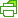Print Friendly

# Mathematics

Below is a list of selected articles. For more articles see sub-categories.

# Solutions of 2-dim Difference Equations

Kyriakos Kefalas

Closed form solutions of 2-dim systems of autonomous difference equations.

# Iterative Abel Functional Equation: f(y + 1) = u∘f(y)

by Kyriakos Kefalas